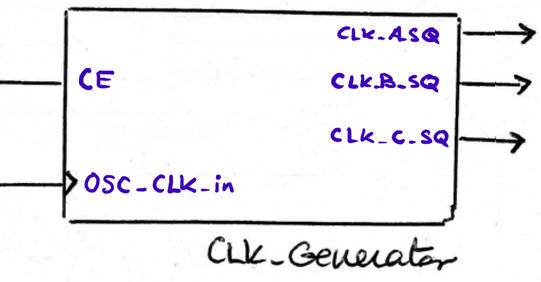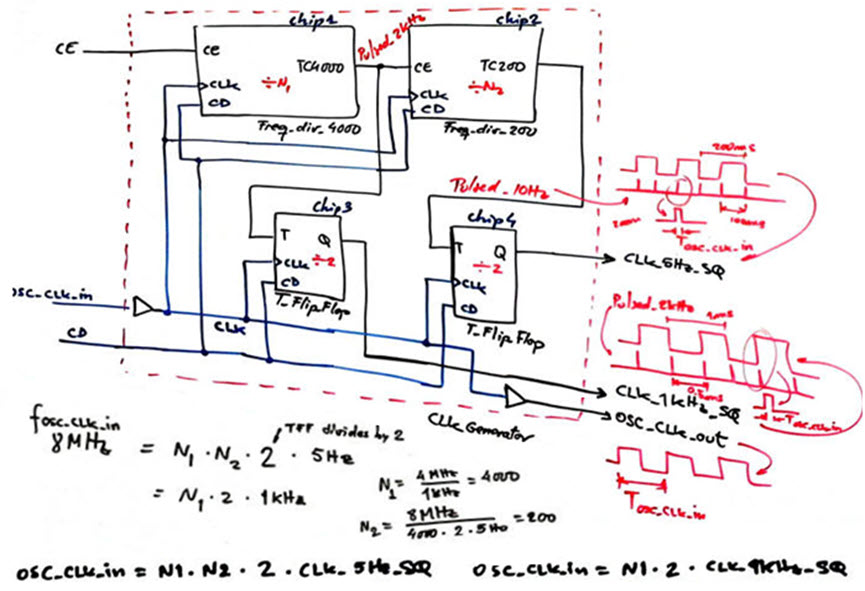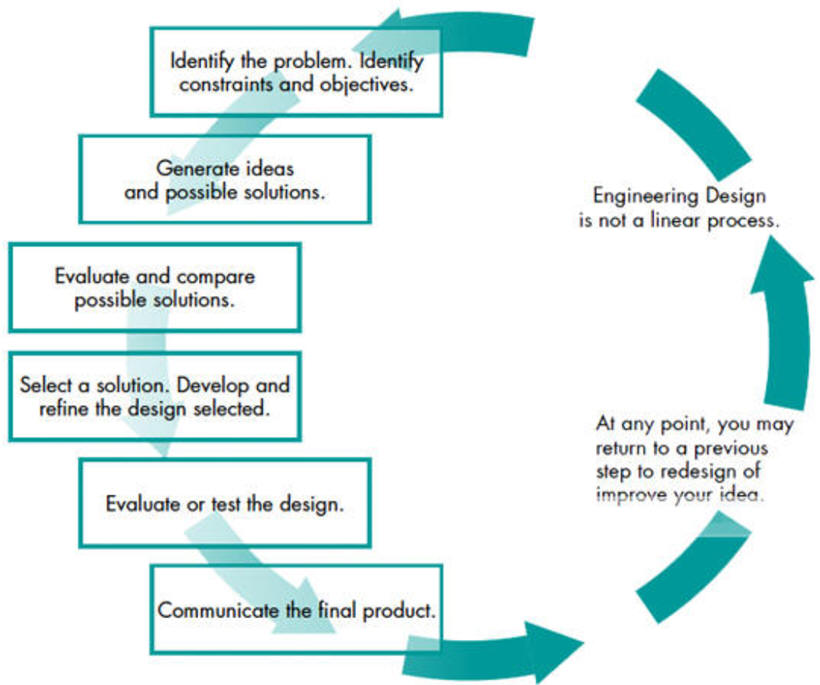﻿ Digital Circuits and Systems - Circuits i Sistemes Digitals (CSD) - EETAC - UPCBachelor's Degree in Telecommunications Systems and in Network EngineeringLecture 2 L8.2: CLK generator design [P8] CLK Generator / timer circuits / applications L9.1 [12/5]

2.8.4. CLK_Generator circuit

1. Specifications of a generic structure

Let's build an example of synchronous CLK_Generator circuit to provide all CLK signals required by a given application. Let us imagine three outputs.Fig. 1. Example entity. Outputs are synchronous with OSC_CLK_in and squared (50% duty cycle waveforms).   The idea is CLK frequency division, where NA is a natural number: FCLK_A_SQ = FOSC_CLK_in/NA

2. Plan C2

The plan will imply using plan C2 consisting of a series of cascaded frequency dividers (Freq_div_Mod) to obtain the pulsed signals and then using T_FF to produce the required squared outputs from the pulsed signals. Generally for common applications, the inicial OSC_CLK_in to which all the derived signals are synchronised is a high frequency crystal quartz .

The idea of dividing frequency by a number is represented in Fig. 2. Imagine the Counter_mod12 at designed using plan Y. If our interest is in the output TC12 and not in the binary code, we can imagine a frequency divder by 12 with a rectangular shape of DC = 12%. Consequently, N = 12, and the next idea is that we can chain the TC12 to a T_FF to solve the problem of squaring this pulsed waveform, at the cost of divided again by 2.Fig. 2. Dividing an input CLK frequency of 1.2 kHz by 24 to generate a 50 Hz synchronous squared output signal.

Thus, the generic plan C2 architecture for CLK_generator is represented in Fig. 3Fig. 3. Proposed generic modular architecture for designing CLK_Generator.

Application example. In this P6 we have designed its CLK_Generator to obtain 1 kHz and 5 Hz signals as follows:Fig. 4. Proposed generic modular architecture for designing CLK_Generator.

Where for instance Freq_div_4000 and Freq_div_200 are easily adapted using plan Y ajusting number of states and size of vector signals.Fig. 5. Plan Y design of the Freq_div_4000 and Freq_div_200.

Explain how to speed up a CLK_Generator testbench when a large amount of time simulation is required. What is the way to shorten the simulation time keeping the circuit structure?

2.8.5. Examples and applications of dedicated processors

2.8.5.1. MM:SS timer (P8) {Timer_MMSS}

The same strategy on charging and discharging capacitors by means of switching networks (logic gates or integrated circuits) is applied in this unit on timer circuits.

2.3.3. and re-triggerable functionality

2.3.3.1. RC timer

2.3.2.3. Integrated circuit 555 timer

Optional acvanced concepts. This is where our CSD introductory course on hardware design and VHDL ends.

This is where an advanced course on digital circuit design should start: the concept of intellectual property (IP), the key to design professional applications targeted to commercial chips.

- Example paper by Okahara, A; Miwa, S.; Kimura, S..

- Framework paper on "The future of semiconductor intellectual property architectural blocks in Europe" link.

- Example of block using intelectual property component libraries.

Another flow from a book explaining the design concept that we have tried to put into practice in our projects.Fig. 6. Typical design cycle in engineering.

Up to this point in the course, you have learned what represents the basics of hardware design of modern digital systems. No doubt that you can continue this subject's content designing with hardware tools and VHDL advanced systems and even microprocessors and microcontrollers. This is the path proposed in most of the on digital systems. However, the chapter 3 of our introductory CSD course will be based on the use of a commercial microcontroller, precisely for repeating some previous projects from the software point of view.